# High School Physics : Understanding Electricity

## Example Questions

### Example Question #1 : Understanding Electricity

What is the maximum power consumption of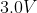portable CD player that draw a maximum of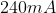of current?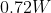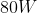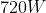Explanation:

The power in a circuit is equal to the current times the voltage.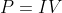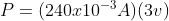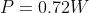### Example Question #941 : High School Physics

The total amount of charge that passes through a wire’s full cross section at any point per unit of time is referred to as

Current

Wattage

Electric potential

Voltage

Current

Explanation:

Current is defined as the amount of charge that passes through a specific area of a wire in a specific interval of time.  It is measured in Amps which are Coulombs per second.

### Example Question #942 : High School Physics

If the resistance in a constant voltage circuit is doubled, the power dissipated by that circuit

Increase by a factor of two

Decrease to one half its original value

Increase by a factor of four

Decrease to one fourth its original value

Decrease to one half its original value

Explanation:

Decrease to one half its original value

The equation for Power isAccording to Ohm’s Law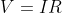We can rearrange this equation for current as this is what is changing in our circuit.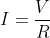We can then substitute this back into the power equation.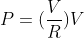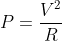If we have the original value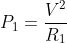and our second value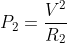We can rearrange both for the voltage and set them equal to each other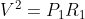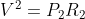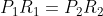So we can substitute this value in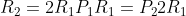And solve for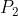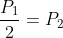Therefore the power would be cut in half.

### Example Question #943 : High School Physics

A current of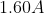flow in a wire.  How many electrons are flowing past any point in the wire per second?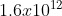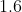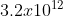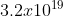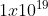Explanation:

The current is a measure of the amount of charge that passes a given point in a certain amount of time.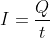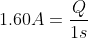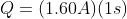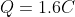To determine how many electrons are passing this point we need to look at the charge of 1 electron and do a conversion.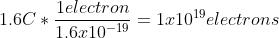### Example Question #944 : High School Physics

The direction of conventional current is taken to be the direction that __________________ .

negative charges would flow

positive charges would flow

positive charges would flow

Explanation:

We often think of current flowing from the top of the battery to the bottom of the battery.  The top of the battery has a higher electrical potential than the bottom of the battery and is associated as being positive.  Charges interact in such a way where like charges repel and opposite charges attract.  Since we think of the charges traveling away from the positive end of the battery and toward the negative end of the battery, this would model the motion of a positive charge (away from positive and toward negative).  Since it is not protons that move through the circuit, but rather electrons.  It is more accurate to describe the flow of electrons from the negative side of the battery to the positive side.

### Example Question #945 : High School Physics

Why might a circuit breaker open if you plug too many electrical devices into a single circuit?

The current becomes too high

A circuit breaker will not “trip” no matter how many electrical devices you plug into the circuit

The resistance becomes too high

The voltage becomes too high

The current becomes too high

Explanation:

When plugin objects into a single circuit, these objects are connected in parallel as each one will receive the 120V from the electrical outlet. However, as additional objects are added, the current is thereby increased. Circuit breakers are designed to trip once the current reaches a maximum load and shuts down the circuit to protect the wires, and the devices that are plugged into the circuit as high current can damage these devices.

### Example Question #946 : High School Physics

A 4.5V battery is connected to a bulb whose resistance is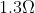. How many electrons leave the battery per minute?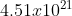electrons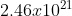electrons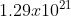electrons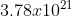electronselectrons

Explanation:

We first need to determine the current coming through the bulb. We can use Ohm’s law to determine this.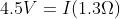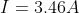Current by definition is the amount of charge per unit time.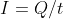We are looking at the number of electrons in one minute which is 60 seconds.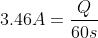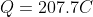We now know the amount of charge passing through in 60 seconds.  We know that the charge of the electron is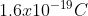.  We can use this to figure out how many electrons are going through.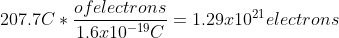### Example Question #947 : High School Physics

Nothing happens when birds land on a power line, yet we are warned not to touch a power line with a ladder. What is the difference?

There is little to no voltage drop between a bird's two feet, but there is a significant voltage drop between the top of a ladder touching a power line and the bottom of the ladder on the ground

Dangerous current comes from the ground only

Birds have extremely high internal resistance compared to humans

Most birds don’t understand the situation

There is little to no voltage drop between a bird's two feet, but there is a significant voltage drop between the top of a ladder touching a power line and the bottom of the ladder on the ground

Explanation:

Electricity travels from the point of highest potential to the lowest potential. We measure the difference between these two potentials as the voltage. Since both of the birds' feet are on the wire, both feet have the same potential. However, if the ladder touches the power line and the bottom of the ladder is on the ground, there is a much higher potential difference (as the ground is at 0 potential).  Therefore the electricity will travel down the ladder!

### Example Question #948 : High School Physics

When a light switch is turned on, the light comes on immediately because

The lightbulb may be old with low resistance. It would take longer if the bulb were new and had high resistance

The electrons coming from the power source move through the initially empty wires very fast

The electricity bill is paid. The electric company can make it take longer when the bill is unpaid

The electrons already in the wire are instantly “pushed” by the voltage difference